### 3. INFLATION

One of the striking features of the CBR fluctuations is that they appear to be noncausal. The CBR fluctuations were largely imprinted at the time of last-scattering, about 300,000 years after the bang. However, there are fluctuations on length scales much larger than 3000,000 light years! How could a causal process imprint fluctuations on scales larger than the light-travel distance since the time of the bang? The answer is inflation, but to see how that works, let's define the problem more exactly.

First consider the evolution of the Hubble radius with the scale factor a (t): (1)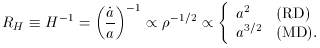(7)

In a k = 0 matter-dominated universe the age is related to H by t = (2/3)H-1, so RH = (3/2)t. In the early radiation-dominated universe t = (1/2)H-1, so RH = 2t.

On length scales smaller than RH it is possible to move material around and make an imprint upon the universe. Scales larger than RH are ``beyond the Hubble radius,'' and the expansion of the universe prevents the establishment of any perturbation on scales larger than RH.

Next consider the evolution of some physical length scale. Clearly, any physical length scale changes in expansion in proportion to a (t).

Now let us form the dimensionless ratio L/ RH. If L is smaller than unity, the length scale is smaller than the Hubble radius and it is possible to imagine some microphysical process establishing perturbations on that scale, while if L is larger than unity, no microphysical process can account for perturbations on that scale.

Since RH = a / dota, anda, the ratio L is proportional to dota, and dotL scales as dotdota, which in turn is proportional to -(+ 3p). There are two possible scenarios for dotL depending upon the sign of+ 3p: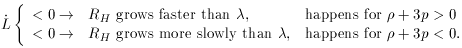(8)

In the standard scenario,+ 3p > 0, RH grows faster than. This is illustrated by the left-hand side of Fig. 5.

For illustration, let us taketo be the present length8 = 8h-1 Mpc, the scale beyond which perturbations today are in the linear regime. The physical length scale, which today is8, was smaller in the early universe by a factor of a (t) / a0, where a0 is the scale factor today. Today, the Hubble radius is H0-1 ~ 3000 h-1 Mpc. Of course, today8 is well within the current Hubble radius. But in the standard picture, RH grows faster than, and there must therefore have been a time when the comoving length scale that corresponds to8 was larger than RH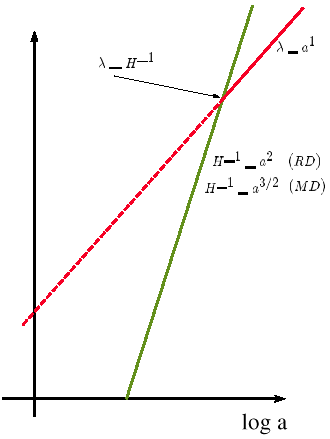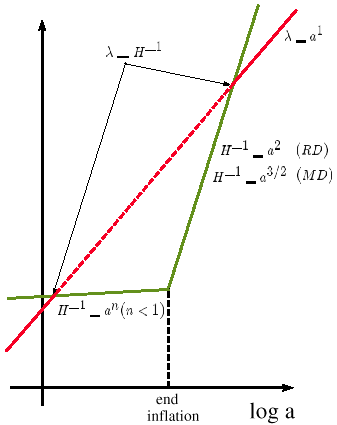Figure 5. Physical sizes increase as a (t) in the expanding universe. The Hubble radius evolves as RH = H-1 = (8G(a) / 3)1/2. In a radiation-dominated or matter-dominated universe (left) any physical length scalestarts larger than RH, then crosses the Hubble radius (= H-1) only once. However, if there was a period of early inflation (right) when RH increased more slowly than a, it is possible for a physical length scale to start smaller than RH, become larger than RH, and after inflation ends become once again smaller than RH. Periods during which the scale is larger than the Hubble radius are indicated by the dotted line.

Sometime during the early evolution of the universe the expansion was such that dotdota > 0, which as we have just seen, requires an unusual equation of state with+ 3p < 0. This is referred to as ``accelerated expansion'' or ``inflation.''

Table 1. Different epochs in the history of the universe and the associated tempos of the expansion rate.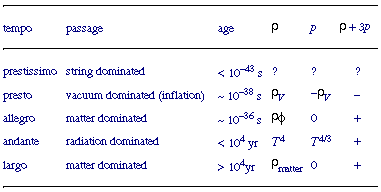Including the inflationary phase, our best guess for the different epochs in the history of the universe is given in Table 1. There is basically nothing known about the stringy phase, if indeed there was one. The earliest phase we have information about is the inflationary phase. As we shall see, the information we have is from the quantum fluctuations during inflation, which were imprinted upon the metric, and can be observed as CBR fluctuations and the departures from homogeneity and isotropy in the matter distribution, e.g., the power spectrum. A lot of effort has gone into studying the end of inflation. It was likely that there was a brief period of matter domination before the universe became radiation dominated. Very little is known about this period after inflation. Noteworthy events that might have occurred during this phase include baryogenesis, phase transitions, and generation of dark matter. We do know that the universe was radiation dominated for almost all of the first 10,000 years. The best evidence of the radiation-dominated era is primordial nucleosynthesis, which is a relic of the radiation-dominated universe in the period 1 second to 3 minutes. The earliest picture of the matter-dominated era is the CBR.

Here, I am interested in events during the inflationary era. The first issue is how to imagine a universe dominated by vacuum energy making a transition to a matter-dominated or radiation-dominated universe. A simple way to picture this is by the action of a scalar fieldwith potential V (). Let's imagine the scalar field is displaced from the minimum of its potential as illustrated in Fig. 6. If the energy density of the universe is dominated by the potential energy of the scalar field, known as the inflaton, then+ 3p will be negative. The vacuum energy disappears when the scalar field evolves to its minimum.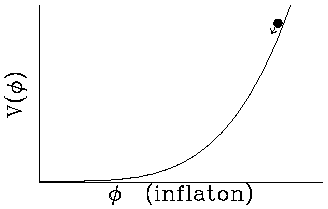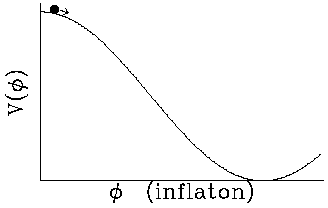Figure 6. Schematic illustrations of the inflaton potential energy. The potential on the left is a ``large-field'' model, where the inflaton field starts large and evolves toward its minimum. The right figure illustrates a ``small-field'' model. A more accurate description of large-field and small-field potential is the sign of the second derivative of the potential: large-field models have V'' > 0 while small-field models have V'' < 0.

If the inflaton is completely decoupled, then it will oscillate about the minimum of the potential, with the cycle-average of the energy density decreasing as a-3, i.e., a matter-dominated universe. But at the end of inflation the universe is cold and frozen in a low-entropy state: the only degree of freedom is the zero-momentum mode of the inflaton field. It is necessary to ``defrost'' the universe and turn it into a ``hot'' high-entropy universe with many degrees of freedom in the radiation. Exactly how this is accomplished is still unclear. It probably requires the inflaton field to be coupled to other degrees of freedom, and as it oscillates, its energy is converted to radiation either through incoherent decay, or through a coherent process involving very complicated dynamics of coupled oscillators with time-varying masses. In either case, it is necessary to extract the energy from the inflaton and convert it into radiation.

(1) Here and throughout the paper ``RD'' is short for radiation dominated, and ``MD'' implies matter dominated. Back